# PROC SQL Joins: A Step-by-Step Guide

This tutorial is designed for beginners who want to get started with PROC SQL Joins. It explains different types of joins and the equivalent data step merge code for these joins. This tutorial includes several examples to help you practice and become proficient in PROC SQL Joins.

Advantages of PROC SQL Joins over Data Step Merging
1. PROC SQL joins do not require sorted tables (data sets), while you need to have two data sets sorted when using Merge Statement
2. PROC SQL joins do not require that common variable have the same name in the data sets you are joining, while you need to have common variable name listed in BY option when using MERGE statement.
3. PROC SQL joins can use comparison operators other than the equal sign (=).
4. PROC SQL can handle many to many relationship well whereas Data Step Merge do not.

## 1. Cross Join / Cartesian product

The Cartesian product returns a number of rows equal to the product of all rows (observations) in all the tables (data sets) being joined. For example, if the first table has 10 rows and the second table has 10 rows, there will be 100 rows (10 * 10) in the merged table (data set).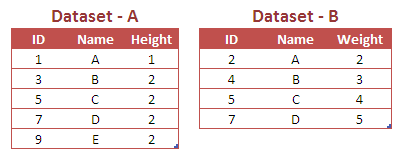Create Sample Datasets

Let's create the two sample datasets that will be used in this tutorial to explain how to use JOINS in SAS.

```Data A;
Input ID Name\$ Height;
cards;
1 A 1
3 B 2
5 C 2
7 D 2
9 E 2
;
run;```
```Data B;
Input ID Name\$ Weight;
cards;
2 A 2
4 B 3
5 C 4
7 D 5
;
run;```

The following code shows how to apply Cartesian Product using PROC SQL in SAS.

```PROC SQL;
Create table dummy as
Select * from A as x cross join B as y;
Quit;```Cartesian or Cross Product
Key takeaways
1. Since the first data set has 5 rows and the second data set has 4 rows, there are 20 rows (5 * 4) in the merged data set.
2. The 'as' keyword (aka alias) is used to assign a table a temporary name.
3. Since the ID values of the first data set is different than the ID values of the second data set, the ID given in the joined data set is misleading.

## 2. Inner Join

The INNER JOIN returns rows common to both tables (data sets). If we select * keyword in the query, the final merged file would have number of columns equal to (Common columns in both the data sets + uncommon columns from data set A + uncommon columns from data set B).Venn Diagram : Inner Join
```PROC SQL;
Create table dummy as
Select * from A as x, B as y
where x.ID = y.ID;
Quit;```Inner Join
Explanation
Since the above case is of type INNER JOIN, it returns values 5 and 7 from the variable ID in the combined table as these two values are common in both the datasets A and B
Another way to write the above code -
```PROC SQL;
Create table dummy as
Select * from A as x inner join B as y
On x.ID = y.ID;
Quit;```

Both the codes produce same result.

Inner Join : Data Step Code
```Data dummy;
Merge A (IN = X) B (IN=Y);
by ID;
If X and Y;
run;```

## 3. Left Join

The LEFT JOIN returns all rows from the left table with the matching rows from the right table.Left Join Venn Diagram
```PROC SQL;
Create table dummy as
Select * from A as x left join B as y
On x.ID = y.ID;
Quit;```Left Join
Explanation
Since the above case is of type LEFT JOIN, it returns all rows from the table (dataset) A with the matching rows from the dataset B.
Left Join : Data Step Code
```Data dummy;
Merge A (IN = X) B (IN=Y);
by ID;
If X ;
run;```

## 4. Right Join

The RIGHT JOIN returns all rows from the right table that do not match any row with the left-hand table, and the matched rows from the left-hand table.Right Join Venn Diagram
```PROC SQL;
Create table dummy as
Select * from A as x right join B as y
On x.ID = y.ID;
Quit;
```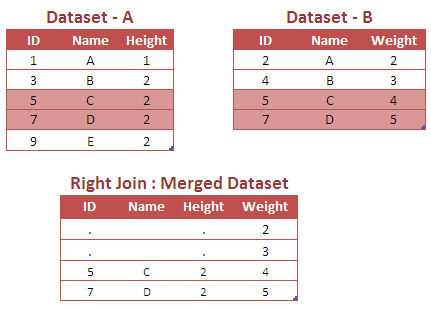Right Join
Note : The right-hand table ID values are missing in the merged table. To add the missing right hand table ID values to a right join, you can use the SQL COALESCE function. The COALESCE function returns the first non-missing argument.
```proc sql;
create table dummy as
select coalesce (x.ID,y.ID) as ID, coalesce (x.name,y.name) as name,height,weight
from a as x right join b as y
on x.id = y.id;
quit;```Right Join with Coalesce
Explanation
Since the above case is of type RIGHT JOIN, it returns all rows from the table (dataset) B with the matching rows from the dataset A.
Right Join : Data Step Code
```Data dummy;
Merge A (IN = X) B (IN=Y);
by ID;
If Y ;
run;```

## 5. Full Join

The FULL JOIN returns all rows from the left table and from the right table.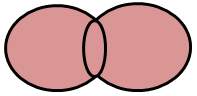Full Join

Key takeaway : The FULL JOIN suffers the same difficulty as the RIGHT JOIN. Namely, the common variable values are lost from the right-hand data set. The COALESCE function can solve this difficulty.

```proc sql;
create table dummy as
select coalesce (x.ID,y.ID) as ID, coalesce (x.name,y.name) as name,height,weight
from a as x full join b as y
on x.id = y.id;
quit;```Full Join with Coalesce
Explanation
Since the above case is of type FULL JOIN, it returns all rows from the table (dataset) A and B.
Full Join : Data Step Code
```Data dummy;
Merge A B;
by ID;
run;```
By default, MERGE statement performs full join so IN variables are not required.
One to Many Relationship : Duplicate Values in Primary KeyJoin : One to Many Relationship

SQL Join will return Cartesian Product if duplicate values are found in primary key (common column). In this example, it returns cartesian product of missing values in the "ID" column. Since dataset A has 3 missing values and dataset B has 1 missing value, there are 3 (3*1) missing values in the merged dataset.

Data Step MERGE statement will return the maximum number of missing values in the primary key in both the tables. In this case, it would return 3 missing values i.e. max(3,1).

Example 2 : One to Many Relationship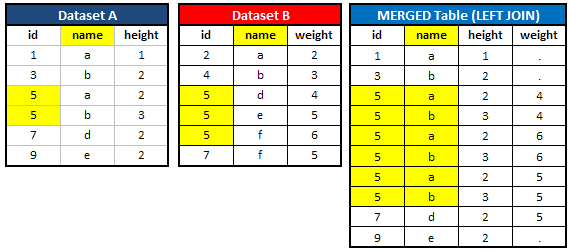PROC SQL : One to Many Relationship
How about six rows of value 5 in the combined table?

When duplicates, PROC SQL returns cartesian product i.e. product of both the tables. In dataset A, we have 2 5s and 3 5s in dataset B. So, it returns (2x3 = 6) 5s in the combined table.

How to refer to permanent library in PROC SQL Joins

In PROC SQL, you can refer to permanent libraries when performing joins by specifying the library and table names - `library_name.table_name`. See the example below.

```PROC SQL;
Create table dummy as
On x.ID = y.ID;
Quit;```
Related Posts
ShareDeepanshu founded ListenData with a simple objective - Make analytics easy to understand and follow. He has over 10 years of experience in data science. During his tenure, he worked with global clients in various domains like Banking, Insurance, Private Equity, Telecom and HR.

45 Responses to "PROC SQL Joins: A Step-by-Step Guide"
1.Its Very Useful... Thanks a lot sharing..!

1.Thank you for stopping by. Glad you found the article useful :-)

2.Well explained! Keep up the good work :-)

1.Thank you for your appreciation :-)

3.Really useful :-)

4.Thanks Deepanshu for sharing this valuable knowledge. Its really a treasure for beginners like me.

5.Well explained..Its really useful especially for beginners. Thank u for sharing.

6.really very helpful ! i have one doubt explain the ten procedures which were used in SQL?

7.Very Helpful and easy to understand
Thanks!

8.Extremely helpful, thank you very much !

9.Moumita ChakrabortyAugust 22, 2015 at 9:08 PM

Really very helpful but I found a small typo. In the full join SQL code wont it be 'full join' instead of 'right join'?

1.Thanks for pointing it out, typo now corrected!

10.1.Glad you found it useful. Cheers!

11.Excellent! good explanation with codes . . . very useful!

12.thank you so much!!

13.Loved your explanation..Very useful for SAS beginners..Keep going

14.very usefull information..

15.Hi Deepanshu,

in all the joins it is mentioned

(Common columns in both the data sets + uncommon columns from data set A + uncommon columns from data set B).

is this true????

1.If you include "*" keyword, it would select variables from both the tables.

16.full join code should be like this
PROC SQL;
Create table dummy as
Select coalesce (x.ID,y.ID) as ID,
coalesce (x.name,y.name) as name,height,weight
from A as x full join B as y
on x.ID = y.ID;
Quit;

1.Thanks for pointing it out. I have corrected the code. Cheers!

17.full join Data Step code should be

Data dummy;
Merge A (IN = X) B (IN=Y);
by ID;
if x=1 or y=1;
run;

1.Please let me know if I am wrong

2.By default, MERGE Statement performs FULL JOIN so "if x=1 or y=1;" is not required. Please let me know incase any query(s) Thanks!

3.The output record will be prepared either from the record of A or B, hence the statement "if x=1 or y=1;" will not be required to mention and it will be by default.

18.Well explained about joins. Very use full for the beginners.

19.Did you read the first paragraph 'Advantages of PROC SQL Joins over Data Step Merging'? That's answer of your first question. Second question is also discussed in the article. If you have duplicates in any of the tables, you would get cartesian product of the duplicate records.

20.Was very useful.!! Well explained.

21.This comment has been removed by the author.

22.What about Outer joins? How it will be prepared using PROC SQL?

23.This is really great website. I'm glad I found it.

24.It helpful for my understanding, Thank you

25.How many datasets can be merged in proc sql (sas 9.4)?

26.I love your site to refer any concept in SAS or SQL

27.28.This comment has been removed by the author.

29.Great, thanks for posting this.

30.excellent explanation

31.Hi Deepanshu,
I appreciate your work and thanks for creating a decent tool. If you'll mention the rules that would be great. Also add interesting scenarios bit logical concepts.
and Syntax of every function statement.
Thanks

32.I dont think ur explaination about inner join is accurate, ud better compare that with Proc SQL outter join.

33.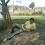Why missing values are coming in right join but not in left join?

34.Great Job!

35.Hi, Deepanshu! It's been years since you last answered to any comments here, so this is a long shot. What if I want to use PROC SQL to join various tables that are listed in sequence? For instance, BIRTHS12, BIRTHS13... BIRTHS21? Is there a way to join tables in a range? [BIRTHS12-BIRTHS21]?

1.In PROC SQL, you can use the %do %to loop to generate a series of join statements for each table from BIRTHS12 to BIRTHS21. Better way to do this via merge statement in SAS (as shown below) -
data want;
merge BIRTHS12-BIRTHS21;
by ID;
run;

Next → ← Prev